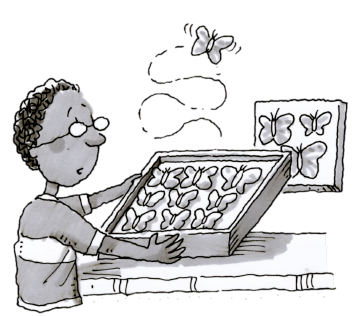### Home > CC2 > Chapter Ch6 > Lesson 6.2.2 > Problem6-66

6-66.

During this chapter, you will use your new solving skills to solve word problems. Think about and use the strategies you already have to answer the questions below.

1.Andy is $4$ years older than Eduardo. If Andy is $x$ years old, write an expression to represent Eduardo’s age.

If Eduardo is $4$ years younger than Andy, how could his age be represented if Andy is $x$ years old?

$x-4$

2. In Eduardo’s collection, the number of butterflies is $12$ more than twice the number of moths. If there are $x$ moths, write an expression to represent the number of butterflies he has.

If twice the number of moths, $x$, can be represented as $2x$, how can the number of butterflies be represented?